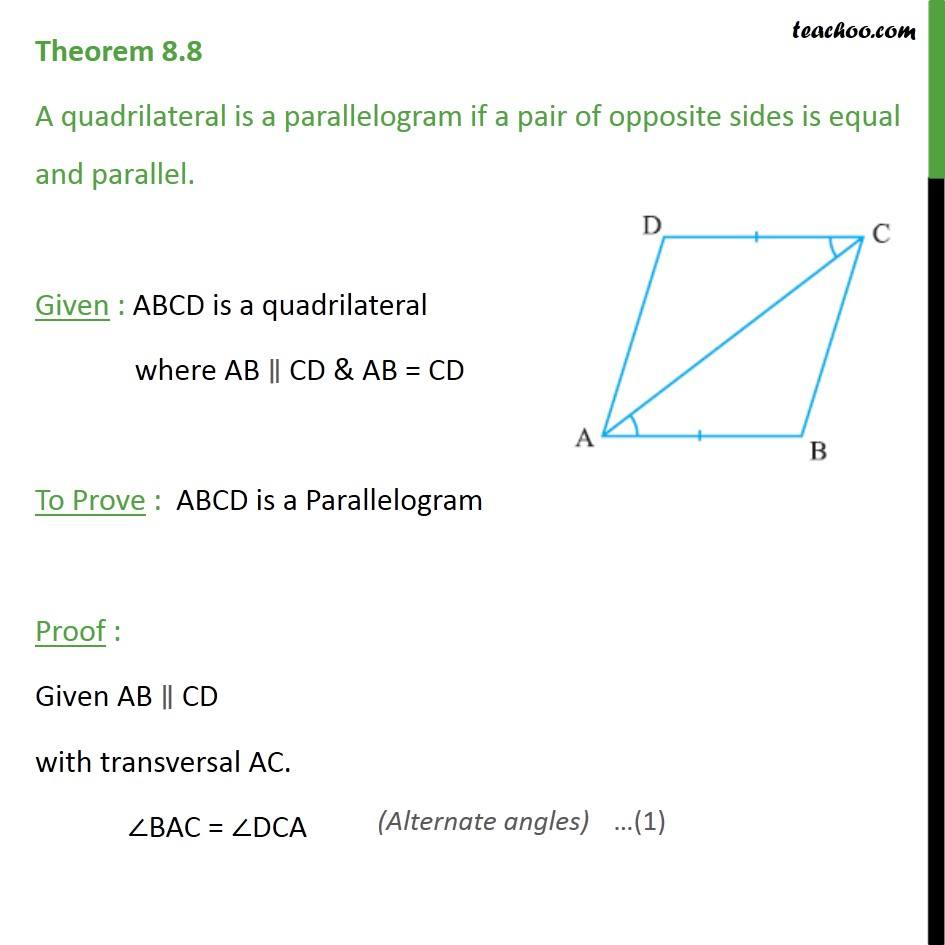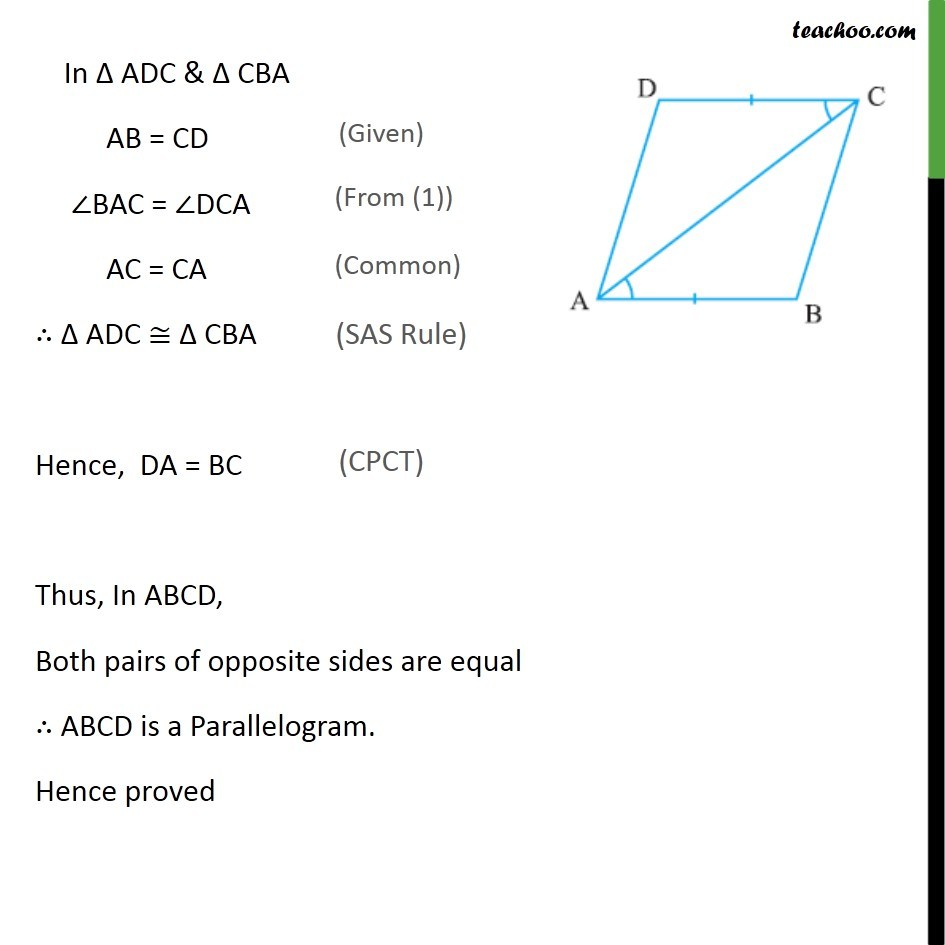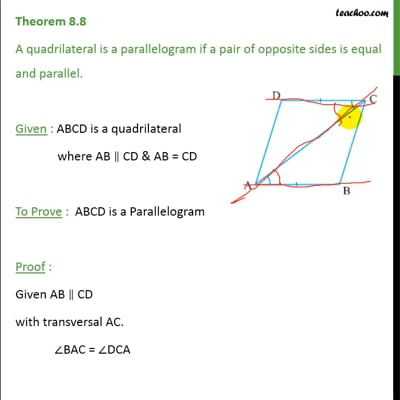Theorems

Chapter 8 Class 9 Quadrilaterals
Serial order wiseThis video is only available for Teachoo black users

Solve all your doubts with Teachoo Black (new monthly pack available now!)

### Transcript

Theorem 8.8 A quadrilateral is a parallelogram if a pair of opposite sides is equal and parallel. Given : ABCD is a quadrilateral where AB CD & AB = CD To Prove : ABCD is a Parallelogram Proof : Given AB CD with transversal AC. BAC = DCA In ADC & CBA AB = CD BAC = DCA AC = CA ADC CBA Hence, DA = BC Thus, In ABCD, Both pairs of opposite sides are equal ABCD is a Parallelogram. Hence proved